# How to Display Negative Numbers in Brackets in Excel

In some situations, like in accounting report or some other reports, we need to add brackets around negative numbers, for example change number format -500 to (-500) or (500). This article will introduce you a quick way to add brackets for negative numbers.

Precondition:

Prepare a table contains some negative numbers.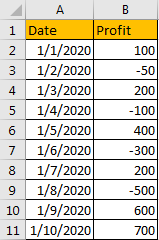## Method: Display Negative Numbers in Brackets

Step 1: Select the list contains negative numbers, then right click to load menu. Click Format Cells on menu.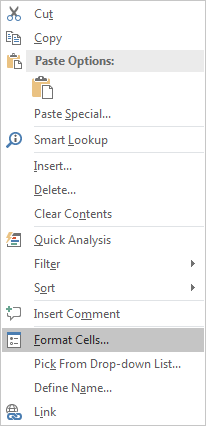Step 2: On Format Cells, under Number tab, click Number in Category list, then in Negative numbers list, select number with brackets. Then click OK to confirm update.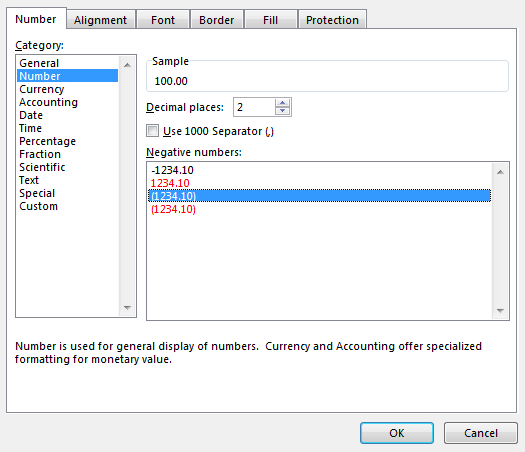Verify that negative numbers are added with brackets.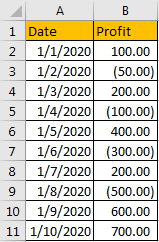If you want to keep number without decimal digits, just reduce decimal places.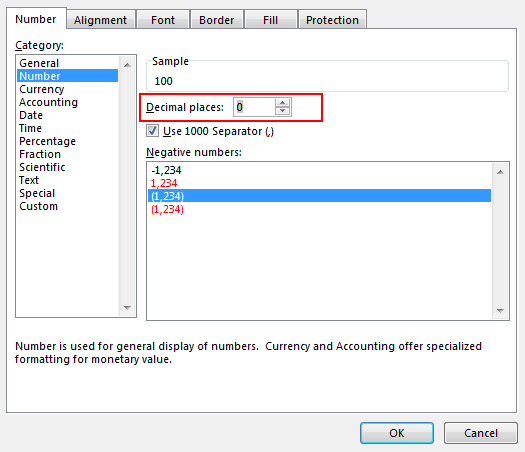Step 3: If you want to keep minus before number, you can select Custom in Category list, then select or enter #,##0_);(-#,##0) in Type field. Then click OK.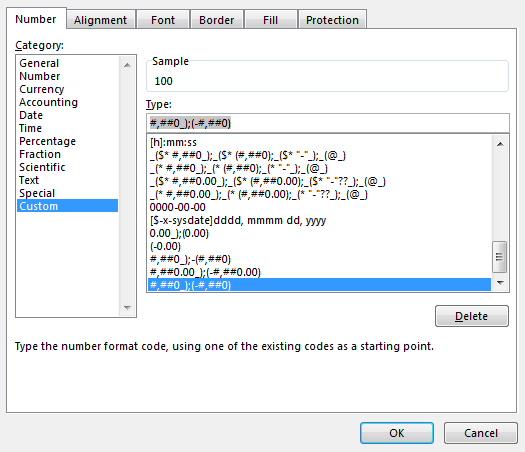Verify that negative numbers are added with brackets properly, and minus are kept for all numbers.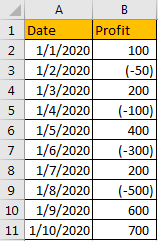If there are decimal digits, you can enter #,##0.00_);(-#,##0.00) in Type.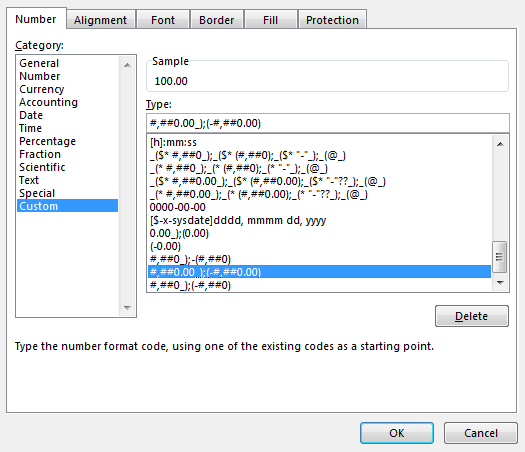Then numbers are displayed with two decimal digits.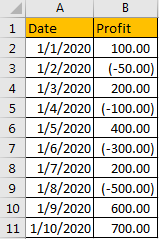Related Posts

If Cell is This Value or That Value

IF function is frequently used in Excel worksheet to return you expect “true value” or “false value” based on the result of logical test. If you want to see if a cell is A or B, and if one of ...

If Value is Greater Than A Certain Value

IF function is frequently used in Excel worksheet to return you expect “true value” or “false value” based on the logical test result. If you want to see if a value in one cell is greater than a specific value, ...

If Cell is Not Blank

IF function is frequently used in Excel worksheet to return you expect “true value” or “false value” based on the result of created logical test. If you want to see if a cell is blank or not, and leave some ...

VBA Macro For VLOOKUP From Another Sheet

In the previous post, you should know that how to fix or remove the #N/A error when using VLOOKUP formula to lookup value from another sheet. And this post will show you how to use VBA code to vlookup data ...

If Cell is Blank

IF function is frequently used in Excel worksheet to return you expect “true value” or “false value” based on the result of created logical test. If you want to see if a cell is blank or not, and leave some ...

If Cell Equals Certain Text String

IF function is frequently used in Excel worksheet to return you expect “true value” or “false value” based on the result of created logical test. If you want to see if cell equals a certain text string like “Win”, you ...

If Cell Contains Either Text1 or Text2

IF function is frequently used in Excel worksheet to return “true value” or “false value” based on the logical test result. If you want to see if cell contains certain substring1 like “abc” or substring2 like “def”, and returns true ...

If Cell Contains Certain Text OR Equals Certain Text

IF cell equals certain text IF function is frequently used in Excel worksheet to return “true value” or “false value” based on the logical test result. If you want to test values to see if they equal certain text like ...

VLOOKUP From Another Sheet Not Working

In the previous post, you should know that how to fix or remove the #N/A error when using VLOOKUP formula to lookup value from another sheet. And this post will show you reasons why your VLOOKUP formula is not working ...

If Cell Begins with One of Three Supplied Characters

If you want to test values to see if they begin with some given specific characters like “x”, ”y”, or “z”, you can create a formula with COUNTIF and SUM functions to return results. EXAMPLE You can see “TRUE” or ...

Sidebar